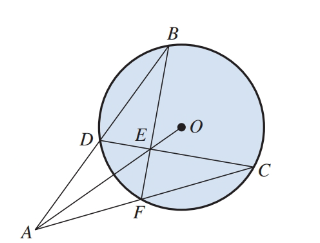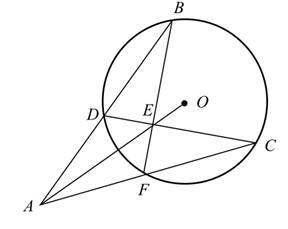Chapter 12.5, Problem 42E

Chapter
Section
Textbook Problem

# Exercises 41–44 refer to Illustration 16. A B ↔ and A C ↔ are secants; C D ¯ and B F ¯ are chords.ILLUSTRATION 16Suppose B C ⌢ = 100 ° and D F ⌢ = 40 ° . Find the measure of ∠ B A C .

To determine

To calculate: The measure of BAC given that BC=100° and DF=40°, where AB and AC are secants; CD¯ and BF¯ are chords.Explanation

Given Information:

A circle in which BC=100° and DF=40°, AB and AC are secants; CD¯ and BF¯ are chords.

Formula used:

The measure of an inscribed angle in a circle is equal to one-half the measure of its intercepted arc.

The exterior angle of a triangle is equal to the sum of interior opposite angles.

Calculation:

Consider the figure,

Here, BC is the intercepted arc of BDC and BDC is an inscribed angle.

Similarly, DF is the intercepted arc of DCF and DCF is an inscribed angle.

Further, the measure of an inscribed angle in a circle is equal to one-half the measure of its intercepted arc.

So, the measure of BDC is equal to one-half the measure of BC and the measure of DCF is equal to one-half the measure of the arc given by DF

### Still sussing out bartleby?

Check out a sample textbook solution.

See a sample solution

#### The Solution to Your Study Problems

Bartleby provides explanations to thousands of textbook problems written by our experts, many with advanced degrees!

Get Started

## Additional Math Solutions

#### Find more solutions based on key concepts